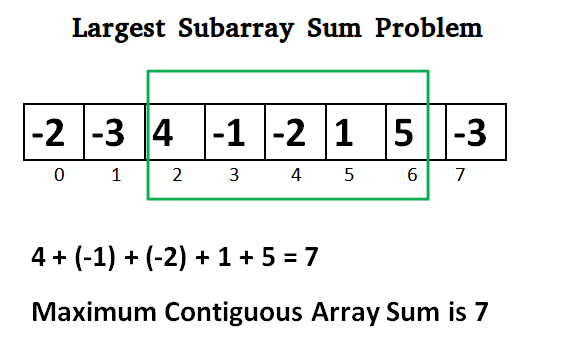# C# Program for Largest Sum Contiguous Subarray

Write an efficient program to find the sum of contiguous subarray within a one-dimensional array of numbers which has the largest sum.## C#

 `// C# program to print largest ` `// contiguous array sum ` `using` `System; ` ` `  `class` `GFG { ` `    ``static` `int` `maxSubArraySum(``int``[] a) ` `    ``{ ` `        ``int` `size = a.Length; ` `        ``int` `max_so_far = ``int``.MinValue, ` `            ``max_ending_here = 0; ` ` `  `        ``for` `(``int` `i = 0; i < size; i++) { ` `            ``max_ending_here = max_ending_here + a[i]; ` ` `  `            ``if` `(max_so_far < max_ending_here) ` `                ``max_so_far = max_ending_here; ` ` `  `            ``if` `(max_ending_here < 0) ` `                ``max_ending_here = 0; ` `        ``} ` ` `  `        ``return` `max_so_far; ` `    ``} ` ` `  `    ``// Driver code ` `    ``public` `static` `void` `Main() ` `    ``{ ` `        ``int``[] a = { -2, -3, 4, -1, -2, 1, 5, -3 }; ` `        ``Console.Write(``"Maximum contiguous sum is "` `+ maxSubArraySum(a)); ` `    ``} ` `} ` ` `  `// This code is contributed by Sam007_ `

Output:

```Maximum contiguous sum is 7
```

Above program can be optimized further, if we compare max_so_far with max_ending_here only if max_ending_here is greater than 0.

## C#

 `static` `int` `maxSubArraySum(``int``[] a, ` `                          ``int` `size) ` `{ ` `    ``int` `max_so_far = 0, ` `        ``max_ending_here = 0; ` ` `  `    ``for` `(``int` `i = 0; i < size; i++) { ` `        ``max_ending_here = max_ending_here + a[i]; ` `        ``if` `(max_ending_here < 0) ` `            ``max_ending_here = 0; ` ` `  `        ``/* Do not compare for all ` `    ``elements. Compare only  ` `    ``when max_ending_here > 0 */` `        ``else` `if` `(max_so_far < max_ending_here) ` `            ``max_so_far = max_ending_here; ` `    ``} ` `    ``return` `max_so_far; ` `} ` ` `  `// This code is contributed ` `// by ChitraNayal `

Please refer complete article on Largest Sum Contiguous Subarray for more details!

My Personal Notes arrow_drop_up

Improved By : nidhi_biet

Article Tags :

Be the First to upvote.

Please write to us at contribute@geeksforgeeks.org to report any issue with the above content.